Courses

Test: Data Handling

10 Questions MCQ Test Mathematics for Class 1 | Test: Data Handling

Description
Attempt Test: Data Handling | 10 questions in 15 minutes | Mock test for Class 1 preparation | Free important questions MCQ to study Mathematics for Class 1 for Class 1 Exam | Download free PDF with solutions
QUESTION: 1

How many Triangles in the figure?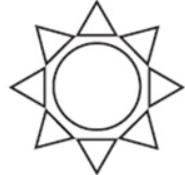Solution: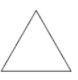A triangle is a polygon with three edges and three vertices. It is one of the basic shapes in geometry.

So, 8 triangle in this figure.

QUESTION: 2

How many apples in the basket?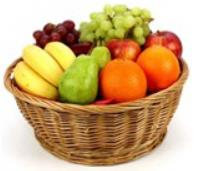Solution: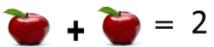QUESTION: 3

How many Triangles in the figure?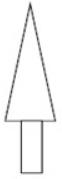Solution:1  triangle in this figure.

QUESTION: 4

How many Triangles in the figure?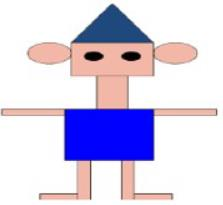Solution:1  triangle in this figure.

QUESTION: 5

How many bananas in the basket?Solution:2+1 = 3
3 Banana In this figure.

QUESTION: 6

How many rectangles in the figure?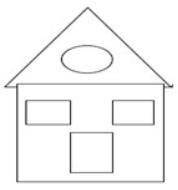Solution: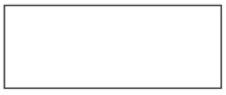A rectangle is a four-sided shape whose corners are all ninety degree angles. Each side of a rectangle is the same length as the one opposite to it.

So, 2 rectangles in this figure.

QUESTION: 7

How many circles in the figure?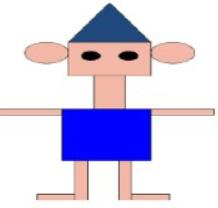Solution: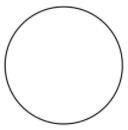A circle is a round shaped figure that has no corners or edges.
In geometry, a circle can be defined as a closed, two - dimensional curved shape.

So, 4 circles in this figure.

QUESTION: 8

How many circles in the figure?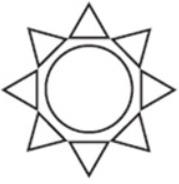Solution:1 circle in this figure.

QUESTION: 9

How many pears in the basket?Solution: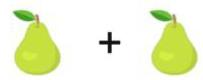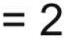QUESTION: 10

How many circles in the figure?Solution:1 circle in this figure.Use Code STAYHOME200 and get INR 200 additional OFF Use Coupon Code

Track your progress, build streaks, highlight & save important lessons and more!

Similar Content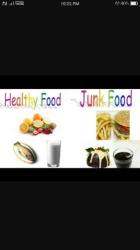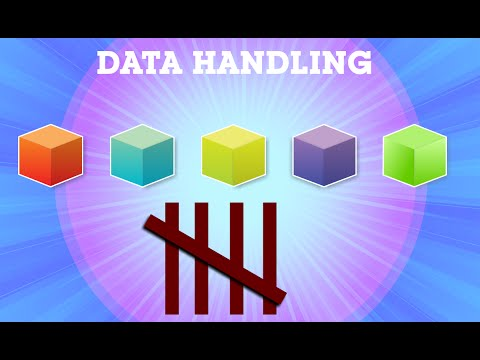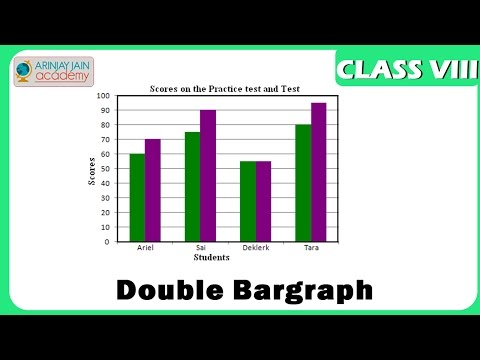Related tests